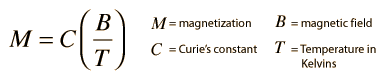# Magnetic Properties of Solids

Materials may be classified by their response to externally applied magnetic fields as diamagnetic, paramagnetic, or ferromagnetic. These magnetic responses differ greatly in strength. Diamagnetism is a property of all materials and opposes applied magnetic fields, but is very weak. Paramagnetism, when present, is stronger than diamagnetism and produces magnetization in the direction of the applied field, and proportional to the applied field. Ferromagnetic effects are very large, producing magnetizations sometimes orders of magnitude greater than the applied field and as such are much larger than either diamagnetic or paramagnetic effects.

The magnetization of a material is expressed in terms of density of net magnetic dipole moments m in the material. We define a vector quantity called the magnetization M by

M = μtotal/V
.

Then the total magnetic field B in the material is given by

B = B0 + μ0M

where μ0 is the magnetic permeability of space and B0 is the externally applied magnetic field. When magnetic fields inside of materials are calculated using Ampere's law or the Biot-Savart law, then the μ0 in those equations is typically replaced by just μ with the definition

μ = Kmμ0

where Km is called the relative permeability. If the material does not respond to the external magnetic field by producing any magnetization, then Km = 1. Another commonly used magnetic quantity is the magnetic susceptibility which specifies how much the relative permeability differs from one.

Magnetic susceptibility χm = Km - 1

For paramagnetic and diamagnetic materials the relative permeability is very close to 1 and the magnetic susceptibility very close to zero. For ferromagnetic materials, these quantities may be very large.

Another way to deal with the magnetic fields which arise from magnetization of materials is to introduce a quantity called magnetic field strength H . It can be defined by the relationship

H = B00 = B/μ0 - M

and has the value of unambiguously designating the driving magnetic influence from external currents in a material, independent of the material's magnetic response. The relationship for B above can be written in the equivalent form

B = μ0(H + M)

H and M will have the same units, amperes/meter.

Ferromagnetic materials will undergo a small mechanical change when magnetic fields are applied, either expanding or contracting slightly. This effect is called magnetostriction.

Index

 HyperPhysics***** Condensed Matter ***** Electricity and Magnetism R Nave
Go Back

# Diamagnetism

The orbital motion of electrons creates tiny atomic current loops, which produce magnetic fields. When an external magnetic field is applied to a material, these current loops will tend to align in such a way as to oppose the applied field. This may be viewed as an atomic version of Lenz's law: induced magnetic fields tend to oppose the change which created them. Materials in which this effect is the only magnetic response are called diamagnetic. All materials are inherently diamagnetic, but if the atoms have some net magnetic moment as in paramagnetic materials, or if there is long-range ordering of atomic magnetic moments as in ferromagnetic materials, these stronger effects are always dominant. Diamagnetism is the residual magnetic behavior when materials are neither paramagnetic nor ferromagnetic.

Any conductor will show a strong diamagnetic effect in the presence of changing magnetic fields because circulating currents will be generated in the conductor to oppose the magnetic field changes. A superconductor will be a perfect diamagnet since there is no resistance to the forming of the current loops.

 Table of magnetic susceptibilities
 Magnetic properties of solids
Index

 HyperPhysics***** Condensed Matter ***** Electricity and Magnetism R Nave
Go Back

# Paramagnetism

Some materials exhibit a magnetization which is proportional to the applied magnetic field in which the material is placed. These materials are said to be paramagnetic and follow Curie's law:All atoms have inherent sources of magnetism because electron spin contributes a magnetic moment and electron orbits act as current loops which produce a magnetic field. In most materials the magnetic moments of the electrons cancel, but in materials which are classified as paramagnetic, the cancelation is incomplete.

 Table of magnetic susceptibilities
 Magnetic properties of solids
Index

 HyperPhysics***** Condensed Matter ***** Electricity and Magnetism R Nave
Go Back